# ForcesPage 1

#### WATCH ALL SLIDES

Slide 1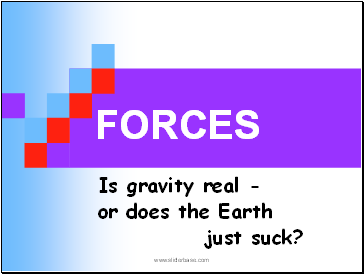FORCES

Is gravity real -

or does the Earth

just suck?

Slide 2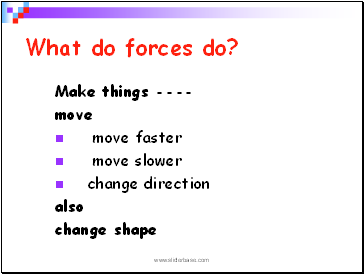## What do forces do?

Make things ----

move

move faster

move slower

change direction

also

change shape

Slide 3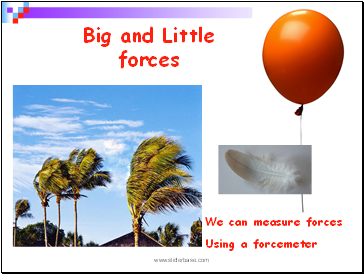Big and Little forces

We can measure forces

Using a forcemeter

Slide 4Sir Isaac Newton

Slide 5Which fruit ?

Slide 6Newton?

Slide 7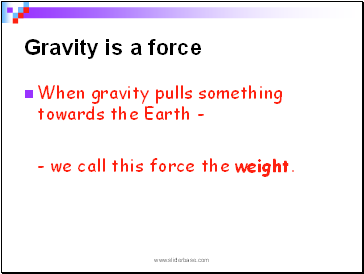## Gravity is a force

When gravity pulls something towards the Earth -

- we call this force the weight.

Slide 8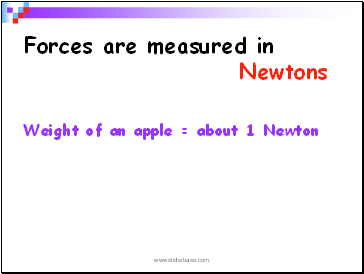## Forces are measured in Newtons

Weight of an apple = about 1 Newton

Slide 9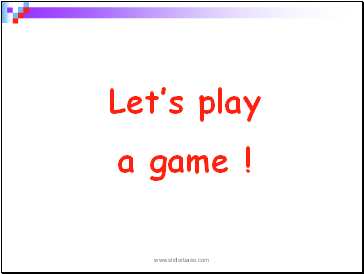Let’s play

a game !

Slide 10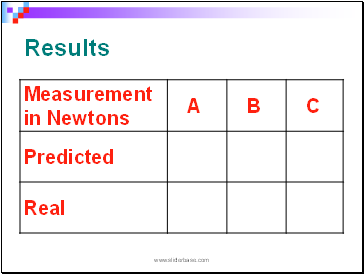Results

Slide 11Forcemeters

Slide 12Now another game!

Slide 13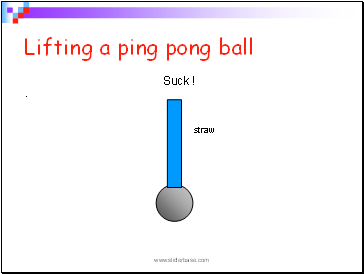Lifting a ping pong ball

.

straw

Suck !

Slide 14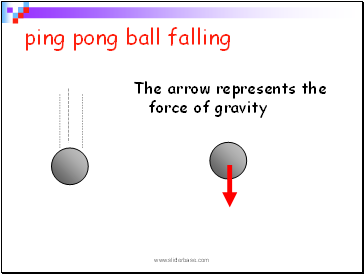ping pong ball falling

The arrow represents the force of gravity

Slide 15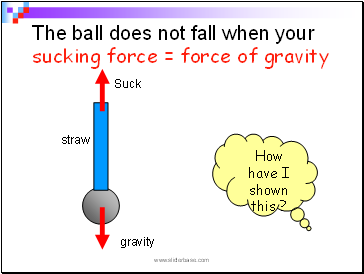The ball does not fall when your sucking force = force of gravity

How have I shown this ?

Slide 16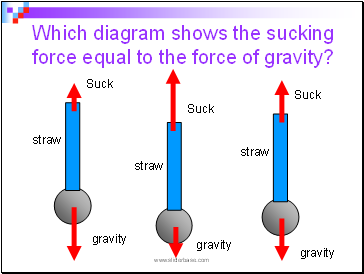Which diagram shows the sucking force equal to the force of gravity?

gravity

gravity

Slide 17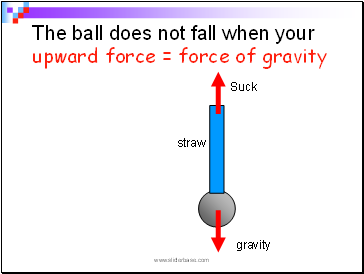The ball does not fall when your upward force = force of gravity

Slide 18Gravity down under

People do not fall off the Earth

Slide 19Go to page:
1  2  3